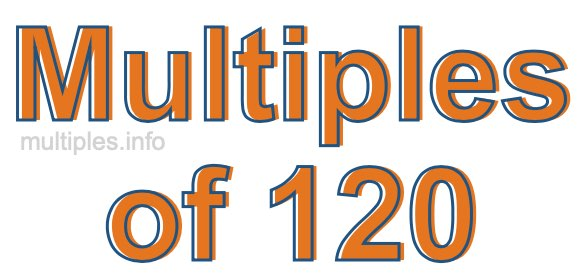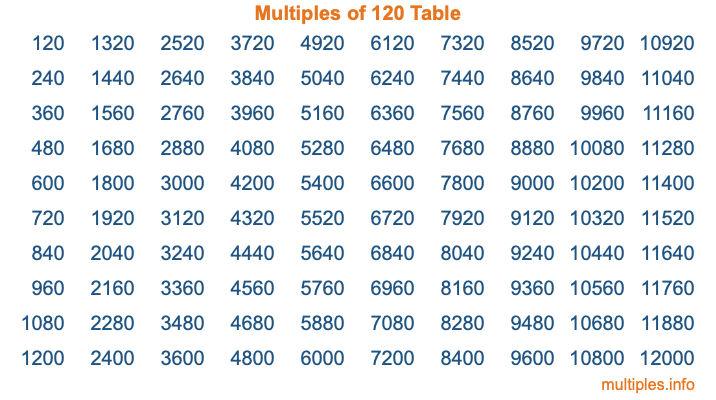Multiples of 120Welcome to the Multiples of 120 page. Here we will first teach you everything you will ever need to know about the multiples of 120, and then give you a study guide summary of everything we taught you to make sure you remember it all. Use this page to look up facts and learn information about the multiples of 120. This page will make you a multiples of one hundred twenty expert!

Definition of Multiples of 120
Multiples of 120 are all the numbers that when divided by 120 equal an integer. Each of the multiples of 120 are called a multiple. A multiple of 120 is created by multiplying 120 by an integer.

Therefore, to create a list of multiples of 120, you start with 1 multiplied by 120, then 2 multiplied by 120, then 3 multiplied by 120, and so on for as long as you want. Thus, the list of the first five multiples of 120 is 120, 240, 360, 480, and 600. To see a larger list of multiples of 120, see the printable image of Multiples of 120 further down on this page. We also have a category where you can choose any nth multiple of 120.

Multiples of 120 Checker
The Multiples of 120 Checker below checks to see if any number of your choice is a multiple of 120. In other words, it checks to see if there is any number (integer) that when multiplied by 120 will equal your number. To do that, we divide your number by 120. If the the quotient is an integer, then your number is a multiple of 120.

Is  a multiple of 120?

Least Common Multiple of 120 and ...
A Least Common Multiple (LCM) is the lowest multiple that two or more numbers have in common. This is also called the smallest common multiple or lowest common multiple and is useful to know when you are adding our subtracting fractions. Enter one or more numbers below (120 is already entered) to find the LCM.

Check out our LCM Calculator if you need more details about the Least Common Multiple or if you need the LCM for different numbers for adding and subtraction fractions.

nth Multiple of 120
As we stated above, 120 is the first multiple of 120, 240 is the second multiple of 120, 360 is the third multiple of 120, and so on. Enter a number below to find the nth multiple of 120.

th multiple of 120

Multiples of 120 vs Factors of 120
120 is a multiple of 120 and a factor of 120, but that is where the similarities end. All postive multiples of 120 are 120 or greater than 120. All positive factors of 120 are 120 or less than 120.

Below is the beginning list of multiples of 120 and the factors of 120 so you can compare:

Multiples of 120: 120, 240, 360, 480, 600, etc.

Factors of 120: 1, 2, 3, 4, 5, 6, 8, 10, 12, 15, 20, 24, 30, 40, 60, 120

As you can see, the multiples of 120 are all the numbers that you can divide by 120 to get a whole number. The factors of 120, on the other hand, are all the whole numbers that you can multiply by another whole number to get 120.

It's also interesting to note that if a number (x) is a factor of 120, then 120 will also be a multiple of that number (x).

Multiples of 120 vs Divisors of 120
The divisors of 120 are all the integers that 120 can be divided by evenly. Below is a list of the divisors of 120.

Divisors of 120: 1, 2, 3, 4, 5, 6, 8, 10, 12, 15, 20, 24, 30, 40, 60, 120

The interesting thing to note here is that if you take any multiple of 120 and divide it by a divisor of 120, you will see that the quotient is an integer.

Multiples of 120 Table
Below is an image of the first 100 multiples of 120 in a table. The table is in chronological order, column by column. The first column has the first ten multiples of 120, the second column has the next ten multiples of 120, and so on.The Multiples of 120 Table is also referred to as the 120 Times Table or Times Table of 120. You are welcome to print out our table for your studies.

Negative Multiples of 120
Although not often discussed or needed in math, it is worth mentioning that you can make a list of negative multiples of 120 by multiplying 120 by -1, then by -2, then by -3, and so on, to get the following list of negative multiples of 120:

-120, -240, -360, -480, -600, etc.

Multiples of 120 Summary
Below is a summary of important Multiples of 120 facts that we have discussed on this page. To retain the knowledge on this page, we recommend that you read through the summary and explain to yourself or a study partner why they hold true.

There are an infinite number of multiples of 120.

A multiple of 120 divided by 120 will equal a whole number.

120 divided by a factor of 120 equals a divisor of 120.

The nth multiple of 120 is n times 120.

The largest factor of 120 is equal to the first positive multiple of 120.

120 is a multiple of every factor of 120.

120 is a multiple of 120.

A multiple of 120 divided by a divisor of 120 equals an integer.

120 divided by a divisor of 120 equals a factor of 120.

Any integer times 120 will equal a multiple of 120.

Multiples of a Number
Here you can get the multiples of another number, all with the same attention to detail as we did for multiples of 120 on this page.

Multiples of
Multiples of 121
Did you find our page about multiples of one hundred twenty educational? Do you want more knowledge? Check out the multiples of the next number on our list!